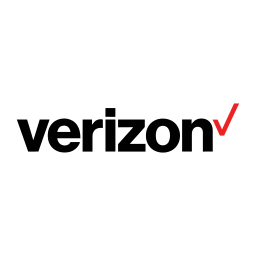# Five S&P 500 High Yield Stocks With Double Digit Earnings Growth

+12.33%
Upside
49.38
Market
55.47
TrefisVZ
Verizon

This article was submitted by Dividend Yield using our Trefis Contributors tool

The S&P 500 index is a popular used tool by investors. Professionals like big insurance player or pension funds allocate their portfolio weighting on indices like the Dow Jones Index and Standard & Poor’s 500. The S&P 500 is a free-float capitalization-weighted index published since 1957 by Standard & Poor’s, a division of McGraw-Hill Companies. I screened the index with its 500 companies by dividend yields over five percent (high yield). The index has sixteen high yields and the highest yield amounted to 8.62 percent, paid by Frontier Communications (FTR). But earnings of the company are expected to decrease by around eight percent for the next year. No good news for a dividend growth investor. Here are the high yield stocks from the index with an expected earnings per share growth of more than 10 percent for the next year. As result, five stocks remained and all have a low beta ratio (stock to market volatility measure).

Here are my favorite stocks:

Relevant Articles

1. Verizon Communications (VZ) has a market capitalization of \$108.09 billion. The company employs 193,900 people, generates revenues of \$110,875.00 million and has a net income of \$10,198.00 million. The firm’s earnings before interest, taxes, depreciation and amortization (EBITDA) amounts to \$29,376.00 million. Because of these figures, the EBITDA margin is 26.49 percent (operating margin 11.62 percent and the net profit margin finally 9.20 percent).

The total debt representing 23.93 percent of the company’s assets and the total debt in relation to the equity amounts to 153.33 percent. Due to the financial situation, a return on equity of 6.45 percent was realized. Twelve trailing months earnings per share reached a value of \$0.85. Last fiscal year, the company paid \$1.98 in form of dividends to shareholders. The earnings per share are expected to grow by 12.05 percent for next year.

Here are the price ratios of the company: The P/E ratio is 45.04, P/S ratio 0.97 and P/B ratio 3.00. Dividend Yield: 5.25 percent. The beta ratio is 0.55.

2. Eli Lilly & Co. (LLY) has a market capitalization of \$45.21 billion. The company employs 38,080 people, generates revenues of \$24,286.50 million and has a net income of \$4,347.70 million. The firm’s earnings before interest, taxes, depreciation and amortization (EBITDA) amounts to \$5,455.60 million. Because of these figures, the EBITDA margin is 22.46 percent (operating margin 22.03 percent and the net profit margin finally 17.90 percent).

The total debt representing percent of the company’s assets and the total debt in relation to the equity amounts to percent. Due to the financial situation, a return on equity of percent was realized. Twelve trailing months earnings per share reached a value of \$3.90. Last fiscal year, the company paid \$1.96 in form of dividends to shareholders. The earnings per share are expected to grow by 14.42 percent for next year.

Here are the price ratios of the company: The P/E ratio is 10.00, P/S ratio 1.85 and P/B ratio 2.89. Dividend Yield: 5.05 percent. The beta ratio is 0.69.

3. Health Care REIT (HCN) has a market capitalization of \$10.46 billion. The company employs 308 people, generates revenues of \$1,421.16 million and has a net income of \$155.04 million. The firm’s earnings before interest, taxes, depreciation and amortization (EBITDA) amounts to \$910.08 million. Because of these figures, the EBITDA margin is 64.04 percent (operating margin 11.01 percent and the net profit margin finally 10.91 percent).

The total debt representing 48.52 percent of the company’s assets and the total debt in relation to the equity amounts to 101.63 percent. Due to the financial situation, a return on equity of 2.02 percent was realized. Twelve trailing months earnings per share reached a value of \$0.62. Last fiscal year, the company paid \$2.84 in form of dividends to shareholders. The earnings per share are expected to grow by 23.58 percent for next year.

Here are the price ratios of the company: The P/E ratio is 87.54, P/S ratio 7.37 and P/B ratio 1.71. Dividend Yield: 5.44 percent. The beta ratio is 0.89.

Take a closer look at the full table of S&P 500 high yields with double digit earnings growth. The average P/E ratio amounts to 10.01 and forward P/E ratio is 10.70. The dividend yield has a value of 5.02 percent. P/B is 2.89 and P/S ratio 1.86. The operating margin amounts to 22.03 percent. The Earnings are expected to grow by 14.42 percent for the next fiscal year.